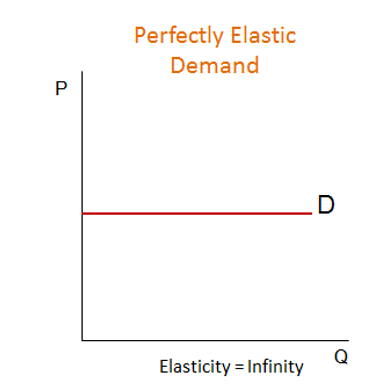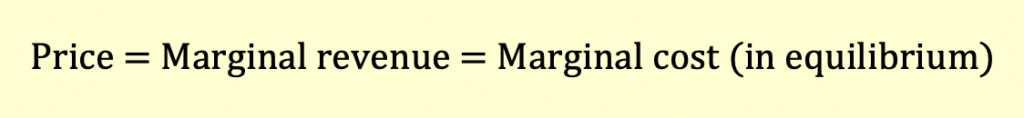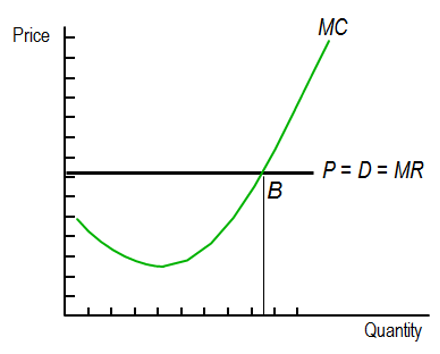# What is Perfect Competition?

##### Elasticity

The firms in perfect competition are price takers. They have no influence on the price of the product. The price is determined by the supply and demand forces.

In perfect competition, each firm faces a perfectly elastic demand curve as shown below. However, the industry will have downward sloping demand curve.##### Optimum Price and Output

The output price and output is calculated by equating the demand and supply function.

The goal of the firm is to maximize profits. A firm maximizes profit when marginal cost (MC) equals marginal revenue (MR), i.e., MC = MR. Till the marginal cost becomes equal to marginal revenue the firm will continue to increase its output. Since each firm is a price taker, marginal revenue is equal to the price of the product (MR = P). So, the marginal revenue curve is the demand curve.The marginal cost first decreases and then increases. The firm will produce quantity Q where marginal cost equals marginal revenue.In the short run, a firm maximizes its economic profit at the quantity where MC = MR. Alternatively, we can say that the profit is maximized at the point where Total revenue – Total cost is maximum.

In the long run, economic profits go to zero because the entry of new firms increases market supply and lowers prices.

### Review of Important Definitions

Total revenue is sales price times quantity sold.

Total costs equal average total costs (ATC) times output level.

Marginal revenue (MR) is the revenue received from an additional unit of output, or the change in total revenue divided by the change in output.

Marginal cost (MC) is the cost of producing an additional unit of output.

Price Elasticity of Demand is the % change in quantity demanded divided by % change in price. Demand can be elastic (E>1), inelastic (E<1), or unitary elastic (E=1). Price elasticity depends on availability of substitutes, how much income consumers spend on the product, and the time period considered.

Get our Data Science for Finance Bundle for just $29$51.